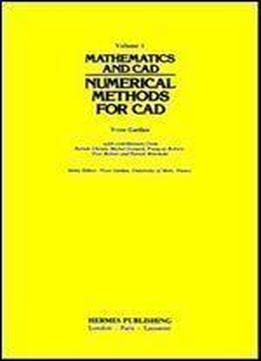# Mathematics And Cad: Numerical Methods For Cad by Yvon Gardan / 1985 / English / PDF

The use of computer-aided design (CAD) systems always involves the introduction of mathematical concepts. It is important, therefore, for any systems designer to have a good grasp of the mathematical bases used in CAD. This book introduces mathematical bases in a general way, so as to allow the reader to understand the basic tools. Based on the-work of leading French researchers, it gives a clear outline of the mathematical concepts employed in setting up a CAD system that uses interactive graphic techniques.

views: 347